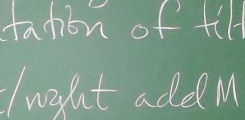BIREP – Representations of finite dimensional algebras at Bielefeld# BIREP Summer School on Koszul Duality

### 10 August – 14 August 2015Koszul Duality

Location: Hotel Waldcafé Jäger, Bad Driburg

Organisers: BIREP research group

Invited speaker: Prof. V. Mazorchuk

## Abstract:

Koszul duality is a concept which occurs in various branches of pure mathematics.

Originating in the study of quadratic algebras it now features prominently in the study of the BGG-category $\mathcal{O}$, in topology (for example, in the work of Goresky, Kottwitz and MacPherson [GKM] on equivariant cohomology), in combinatorics (e.g. Reiner's talk [Re]), for categories of polynomial functors (see the work of Touzé [To] and of Krause [Kr]), and dg-categories (e.g. Keller [Ke]).

These more general forms of Koszul duality originate from the study of quadratic and Koszul algebras. In general, a Koszul ring is a non-negatively graded ring $A=A_0 \oplus A_1 \oplus \ldots$ such that

1. $A_0$ is a semi-simple $A$-module
2. $A_0$ admits a graded projective resolution $P^\ast$ such that $P^i$ is generated in degree $i$.
This simple definition implies a close relation between the graded rings $A=A_0 \oplus A_1 \oplus \ldots$ and $E(A)=Ext^{\ast}(A_0,A_0)$. If $A$ is Koszul and the homogeneous components of $A$ are finitely generated over $A_0$, then $E(E(A))=A$ and the algebras satisfying these conditions share many nice properties. For example, under suitable finiteness assumptions there is a derived equivalence relating $A$ and $E(A)$.
A well-known and helpful introduction to these ideas is given in the Beilinson-Ginzburg-Soergel paper [BGS]. They show that Koszul algebras often arise as Ext-algebras of perverse sheaves on sufficiently nice spaces, giving many more examples.

The prototype and the inspiration for most of the theory is the following example due to Bernstein, Gelfand, and Gelfand [BGG]: let $A=k[x_1, ..., x_n]$ be a polynomial ring. Then $E(A)$ is the exterior algebra on n generators and the BGG-correspondence is a derived equivalence relating $A$ and $E(A)$. The general theory of Koszul algebras explains and generalises this equivalence. The theory also extends to dg-algebras, dg-categories, and their derived categories following the work of several authors e.g., Beilinson-Ginzburg-Schechtmann [BGSc], Beilinson-Ginzburg-Soergel [BGS], and Keller [Ke].

The aim of the school will be to understand and illustrate Koszul duality in several of the incarnations mentioned above. Beginning with the classical theory of Koszul algebras, the original concepts will be introduced and several examples given. In particular, Koszul algebras arising from combinatorics, perverse sheaves on flag varieties, and from Schubert varieties will be discussed. The differential graded point of view will then be discussed, explaining Koszul duality in terms of generators for triangulated categories and relations to exceptional sequences. Brief overviews of Koszul duality in other areas of interest will also be given.

There will be a series of 3 talks given by Prof. V. Mazorchuk.

### Financial Support

Accomodation and meals are covered by the DFG CRC 701 "Spectral Structures and Topological Methods in Mathematics". Travel costs cannot be reimbursed.

### Location

The summer school takes place at the Hotel Waldcafé Jäger, Waldstraße 1, 33014 Bad Driburg. The hotel is in walking distance from the train station of Bad Driburg.

The seminar room in the hotel is equipped with a reasonably sized whiteboard, a beamer and an overhead projector.

Participants will be accommodated in double-rooms.

# Contact

Please contact the organisers for any questions concerning the summer school.